【单选题】求lnx的不定积分为（）
A.
1/x+C
B.
lnx-1+C
C.
xlnx+C
D.
x(lnx-1)+C【多选题】A node in a red-balck tree could be红黑树节点的颜色有
A.
red 红
B.
black 黑
C.
yellow 黄
D.
blue 蓝
E.
green 绿
F.
purple 紫
【多选题】被称为中国新四大发明的有哪些？
A.

B.

C.

D.

E.

F.

【单选题】What does “subtle” mean there?
A.
evident
B.
clear
C.
not obvious
D.
apparent
【单选题】What does “turn out” imply?
A.
The reason for doing something.
B.
Something happens in that way or has the result the consequence of something.
C.
The potential risk of doing something.
D.
The original plan of doing something.
【单选题】What's unique about red-black trees compared to AVL trees? 红黑树相比于AVL树的特点是：
A.
The balance factor of each node falls within [-1, 1] 每个节点的平衡因子的绝对值不超过1
B.
It is a balanced binary search tree 是平衡二叉搜索树
C.
The search time is O(lgn) 支持O(lgn)时间的查找
D.
The topology changes no more than O(1) after each insertion/deletion每次插入/删除后拓扑结构的变化不超过O(1)
【单选题】You may choose one or more than one correct answer(s).1. Why is the student coming for the teacher?
A.
He quarreled with his classmates.
B.
He was stressed about the college entrance examination.
C.
He missed several classes due to illness
D.
He was often stressed when he faced an academic problem.
【多选题】好的选题从哪里来？
A.

B.

C.

D.

E.

【单选题】下列集合中，有最大值的集合是 ( ).
A.
$\{x|x\in (0,1),x\in Q\}$
B.

C.

D.
$\{x\in R|x^2-2x-3 < 0\}$
【单选题】设集合$A=[-1,1], B=(0,2)$，则$A\backslash B =$ ( ).
A.
$[-1,0]$
B.
$[-1,0)$
C.
$(-\infty ,0]$
D.
$( - \infty ,0] \cup [2, + \infty )$
【单选题】设X服从泊松分布，且已知P(X=1)=P(X=2),则P(X=4)=
A.
$0.0304$
B.
$0.0902$
C.
$0.0718$
D.
$0.0625$
【单选题】若$a,b\in R$, 则$\max\{a,b\}$和$\min\{a,b\}$分别是 ( ).
A.
$\frac{{a + b + |a - b|}}{2},\frac{{a + b - |a - b|}}{2}$
B.
$\frac{{a - b + |a - b|}}{2},\frac{{a + b - |a - b|}}{2}$
C.
$\frac{{a + b - |a - b|}}{2},\frac{{a - b + |a - b|}}{2}$
D.
$\frac{{a + b + |a - b|}}{2},\frac{{a - b + |a - b|}}{2}$
【单选题】What is a 'double red' in a red-black tree? 在红黑树中，何为双红缺陷：
A.
the root is red 根节点为红色
B.
both the root and the external nodes are red 根节点和外部节点都为红色
C.
both a parent and its child are red 相邻的两个父子节点都为红色
D.
there are two red nodes in the tree 树中有两个红色节点
【单选题】There seemed to be an _______ supply of champagne at the wedding.
A.
irrelevant
B.
irritable
C.
inexhaustible
D.
irresistible
【单选题】Traveling is _______ more comfortable now than it used to be.
A.
finitely
B.
infinitely
C.
eternally
D.
long-lastingly
【单选题】What does “insomnia” mean?
A.
Lack of vitality.
B.
Lack of sleep.
C.
Loss of interest in food.
【单选题】、用泊松分布作近似计算
A.
$1-1.8e^{-0.8}$
B.
$1-0.8e^{-0.8}$
C.
$1-e^{-0.8}$
D.
$1-2.12e^{-0.8}$
【单选题】接4题，输入电阻Ri将_____，
A.

B.

C.

【单选题】How does the topology changes when fixing a double red and the uncle node u is red.当叔父节点u为红色时，修正双红缺陷导致的红黑树拓扑结构的变化为：
A.
no change 没有变化
B.
it changes no more than O(1) 有变化，但是不超过O(1)
C.
it changes no more than O(lgn) 有变化，但是不超过O(lgn)
D.
it changes no more than O(n) 有变化，但是不超过O(n)
【单选题】函数$y = \frac{1}{{[x + 1]}}$(其中 $[x+1]$是取整函数)的定义域为 ( ).
A.
$(-\infty,-1)$
B.
$[0,+\infty)$
C.
$( - \infty , - 1) \cup [0, + \infty )$
D.
$( - \infty , - 1] \cup (0, + \infty )$
【单选题】谁能帮我读文献？
A.
Pubmed
B.
F1000
C.
Scifinder
【单选题】接4题，输出电阻Ro将_____；
A.

B.

C.

【单选题】函数$y = |x| + |x - 1| - |4 - 2x|$的最小值和最大值分别是 ( ).
A.
$-4,\quad 3$
B.
$-3, \quad 3$
C.
$-3, \quad 4$
D.
$-4, \quad 4$
【单选题】程序编译时出现以下提示，是_____。[Warning] incompatible implicit declaration of built-in function 'printf'
A.

B.

C.

D.

【单选题】设非负实数$x,y$满足方程$(1 - x){y^2} = {x^3}$. 若$x = \frac{{{t^2}}}{{1 + {t^2}}} (t>0)$, 则 ( ).
A.
$y = \frac{{{t^3}}}{{1 + {t^2}}}$
B.
$y = \frac{{{t^3}}}{{{{(1 + {t^2})}^2}}}$
C.
$y = \frac{t}{{1 + {t^2}}}$
D.
$y = \frac{t}{{{{(1 + {t^2})}^2}}}$
【单选题】接4题，输入电阻将_____，
A.

B.

C.

【单选题】、$\sigma$的值为？
A.
2
B.
$\sqrt{2}$
C.
$\frac{1}{\sqrt{2}}$
D.
$\frac{1}{2}$
【单选题】接4题，电压放大倍数将_____，
A.

B.

C.

【单选题】This is a splay tree. What is the result after accessing node v and performing a two-layer splay?上图是伸展树，其中节点v刚被访问过，双层伸展后的结果是：
A.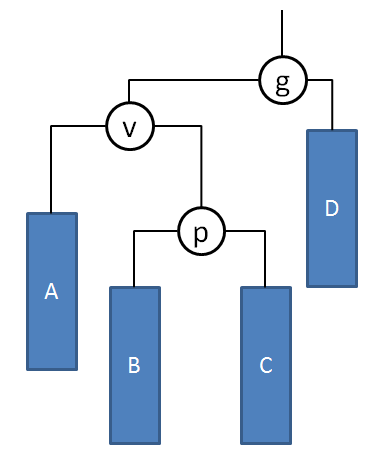B.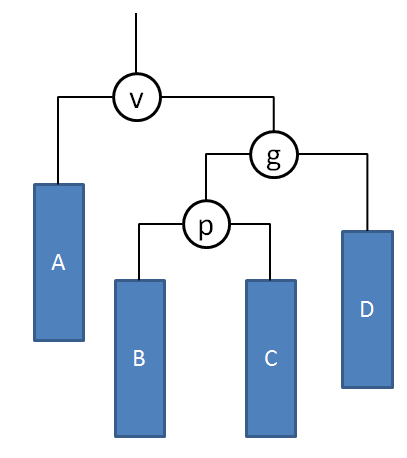C.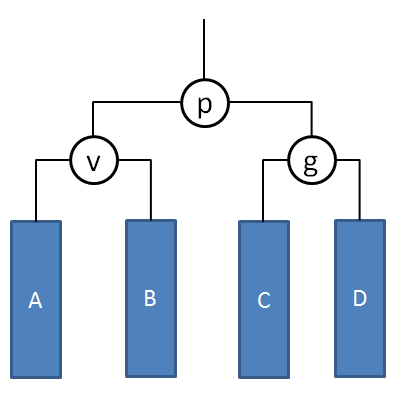D.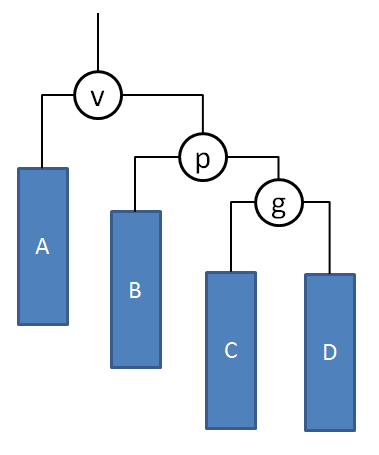【单选题】已知函数$f(x)=1-x^2, x \leq 0 , f(x)= x + 2, x >0$，则$f(x)$ 在$x=0$ 点的单侧连续性
A.

B.

C.

D.

【单选题】设$X\sim N(3,4)$,求P{2 < X ≤ 5}
A.
$\Phi(1)+\Phi(0.5)$
B.
$\Phi(1)-\Phi(-0.5)$
C.
$\Phi(0.5)+\Phi(0.25)$
D.
$\Phi(0.5)-\Phi(-0.25)$
【单选题】This is a splay tree. What's the result after inserting a node 1 and performing a two-layer splay? 在以上伸展树中插入节点1并经过双层伸展后的结果是
A.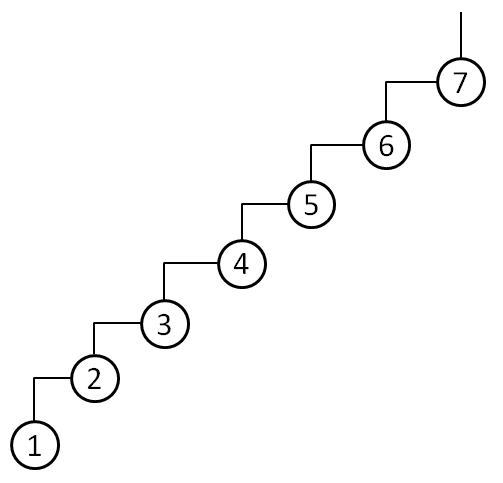B.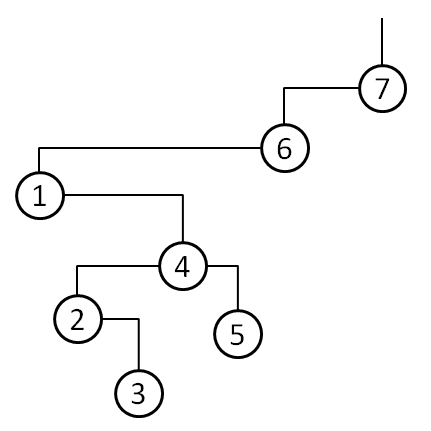C.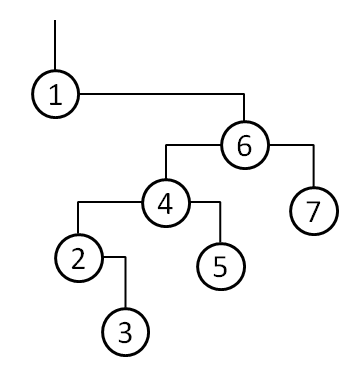D.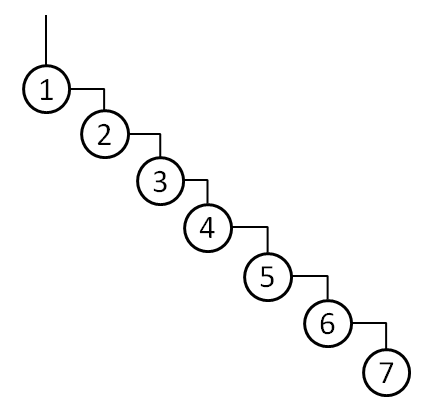【单选题】Which statement regarding (2, 4)-trees is incorrect? 关于(2, 4)-树，下列命题不正确的是：
A.
(2, 4)-trees are equivalent to red-black trees (2,4)-树等价于红黑树
B.
Each internal node has no more than 4 branches 每个内部节点的分支数不超过4
C.
Each internal node has no less than 2 branches 每个内部节点的分支数不小于2
D.
Each node has exactly 3 keys except for the root 除了根节点外，每个内部节点都恰好包含3个关键码
【单选题】以下函数在$x=0$连续的是
A.
$y=sgn x$
B.
$y=\frac{1}{x}$
C.
$y=[x], 其中 [x]$ 是取整函数
D.
$y=sin x$
【判断题】接1题，减小
A.

B.打开"刷刷题APP"，使用更方便